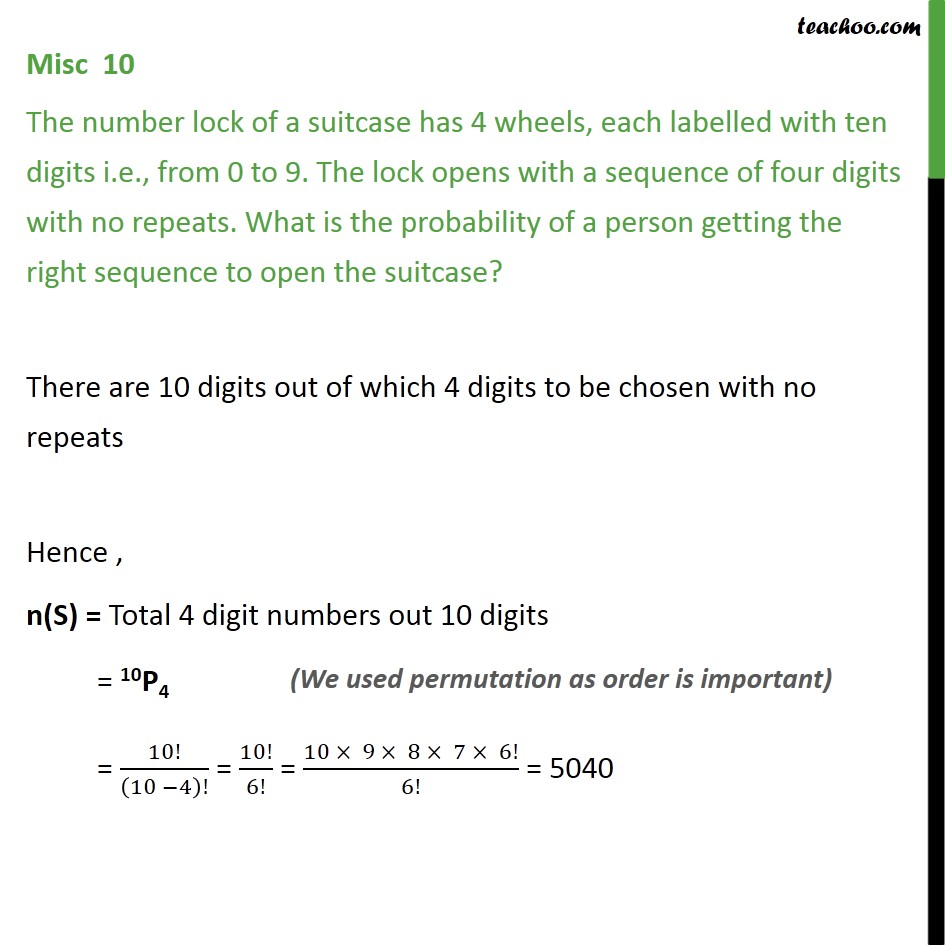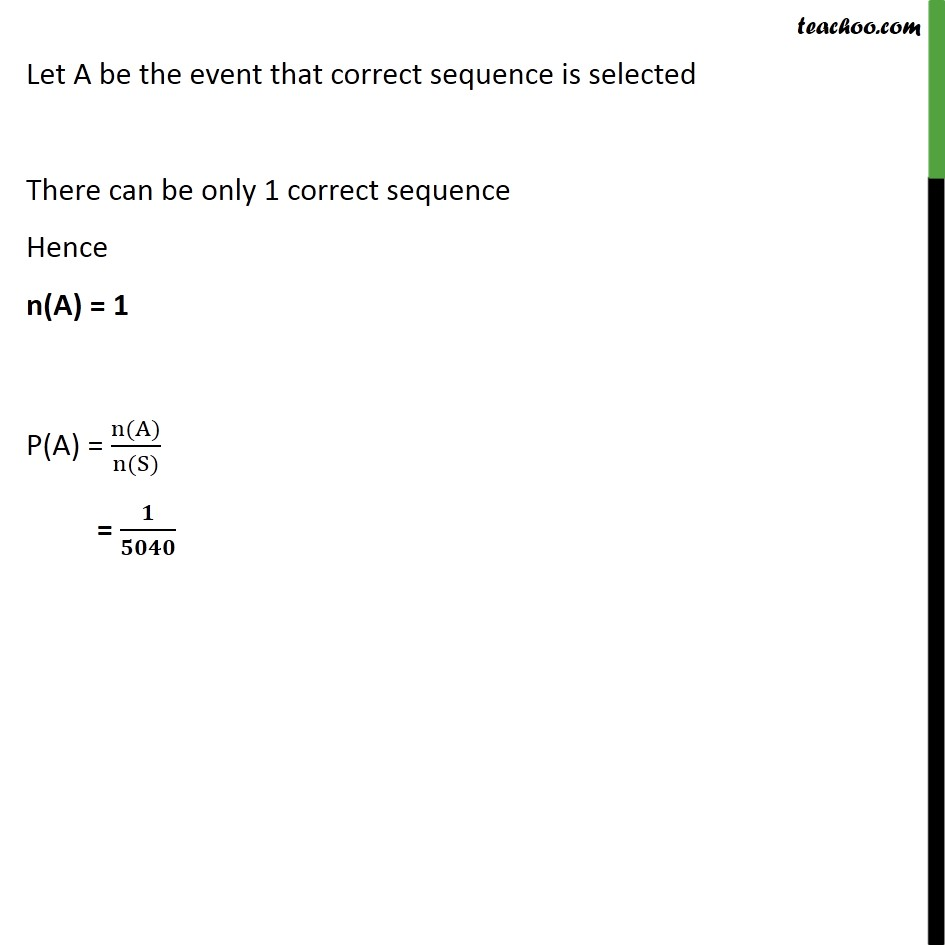Miscellaneous

Chapter 14 Class 11 Probability
Serial order wiseLearn in your speed, with individual attention - Teachoo Maths 1-on-1 Class

### Transcript

Misc 10 The number lock of a suitcase has 4 wheels, each labelled with ten digits i.e., from 0 to 9. The lock opens with a sequence of four digits with no repeats. What is the probability of a person getting the right sequence to open the suitcase? There are 10 digits out of which 4 digits to be chosen with no repeats Hence , n(S) = Total 4 digit numbers out 10 digits = 10P4 = 10!﷮ 10 −4﷯!﷯ = 10!﷮6!﷯ = 10 × 9 × 8 × 7 × 6!﷮6!﷯ = 5040 Let A be the event that correct sequence is selected There can be only 1 correct sequence Hence n(A) = 1 P(A) = n(A)﷮n(S)﷯ = 𝟏﷮𝟓𝟎𝟒𝟎﷯